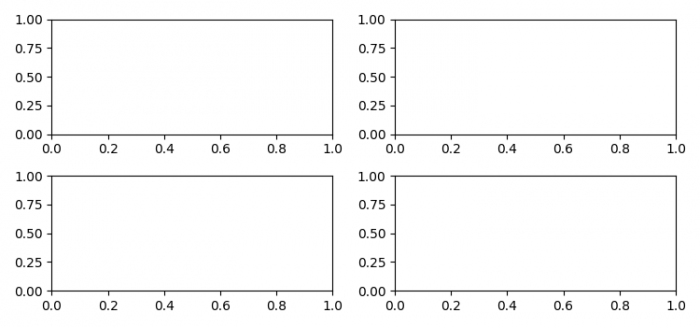# Manipulation on vertical space in Matplotlib subplots

MatplotlibPythonData Visualization

To manipulate on vertical space in Matplotlib subplots, we can use hspace=1 in subplots_adjust() method without tight plot layout.

## Steps

• Set the figure size and adjust the padding between and around the subplots.
• Create x and y data points using numpy.
• Create a figure and a set of subplots with 4 indices.
• To adjust the vertical space, we can use hspace=1.
• To display the figure, use show() method.

## Example

import numpy as np
import matplotlib.pyplot as plt

plt.rcParams["figure.figsize"] = [7.50, 3.50]
plt.rcParams["figure.autolayout"] = True

x = np.linspace(0, 2 * np.pi, 400)
y = np.sin(x ** 2)

fig, ((ax1, ax2), (ax3, ax4)) = plt.subplots(nrows=2, ncols=2)
plt.show()